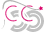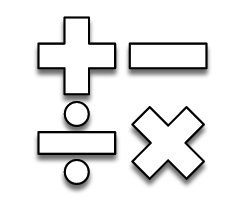Part of The# Maths

Mathematics is an important part of understanding our world, one that enriches our
lives. Thus, children must be given every opportunity to become capable
mathematicians, able to use maths to solve problems and to recognise the beauty of
mathematics.At Fulbridge Academy, we recognise that mathematical understanding is built upon a
foundation of number sense and spatial awareness. To this end, our curriculum
prioritises children’s interaction with manipulatives and their ability to represent their
understanding visually. As their mathematical knowledge and skills develop, children
continue to use concrete manipulatives and visual representations to support their
knowledge of mathematical concepts, progressing towards eventual abstract
understanding. In addition, children’s mathematical vocabulary is systematically
developed to ensure that they can articulate their understanding.

Every child at Fulbridge Academy is supported to learn the key facts of arithmetic,
such as number bonds and multiplication & division facts. These key facts are one
component of the development of mathematical fluency through which children can
choose the most efficient means to complete calculations and solve problems.
Mathematical reasoning is an essential component of all maths lessons, and children
are supported to spot patterns, suggest examples and to explain their understanding
of the mathematics being learned.

Children’s understanding is developed systematically and teachers’ ongoing assessment of children’s learning underpins their teaching and ensures that every child fulfils their potential.

## Reception

• Develop a strong grasp of spatial awareness, including an understanding of patterns of objects, actions and sounds

• Count within 5, including accurate 1:1 counting with objects, an understanding of cardinality and counting of non-tangible objects (e.g. sounds)

• Perceptually subitise (i.e. recognise amount rapidly without counting) groups of objects up 5

• Sort, classify and compare objects based on their characteristics or the number of objects

• Understand the concept of zero in concrete terms as representing absence

• Begin to learn number bonds from 2-5, making use of manipulatives and visual representations

• Order times in the day and recognise the relative lengths of particular units of time (e.g, day, hour)

• Count within 10, including accurate 1:1 counting with objects, an understanding of cardinality and counting of non-tangible objects (e.g. sounds)

• Conceptually subitise (i.e. recognise amount rapidly without counting but using some knowledge of number bonds) groups of objects up to 10, including those shown in a pair structure and a 5-and-a-bit structure

• Understand addition in aggregation and augmentation contexts

• Understand subtraction in partition and reduction contexts

• Double and halve numbers inside 10

• Begin to learn number bonds from 6-10, making use of manipulatives and visual representations

• Count within 20, including accurate 1:1 counting with objects, an understanding of cardinality and counting of non-tangible objects (e.g. sounds); understand that numbers 11-19 can be seen as 10-and-a-bit as an introduction to an understanding of place value

• Recognise common 2D and 3D shapes in different orientations

• Compare and define length, mass and capacity using adjectival, comparative and superlative language (e.g. heavy, heavier, heaviest; long, longer, longest); use non-standard measurements to measure length, mass and capacity

## Year 1

• Count and understand numbers within 100, including counting in 2s, 5s and 10s

• Recognise and using money

• Know the days of the week and months of the year

• Undertake addition and subtraction inside 100

• Fluently use of number bonds inside 20

• Understand the concept of length, mass and capacity, including some standard units

• Recognise and define basic 2D and 3D shapes

• Find basic fractions of amounts

• Understand basic position and direction, including turning

• Begin to tell the time on an analogue clock

• Understand multiplication as repeated addition and division as repeated subtraction (grouping)

## Year 2

• Develop awareness of place value up to 100, including partitioning numbers less than 100 in a variety of ways

• Compare and order numbers up to 100

• Tell the time on an analogue clock to the nearest five minutes

• Develop use of equipment to measure length, mass, capacity and temperature

• Add and subtract numbers inside 100 using a number line, leading to some mental calculation

• Solve basic addition and subtraction problems, including word problems

• Solve problems involving money

• Recognise and define 2D and 3D shapes and understand basic symmetry

• Solve basic problems relating to multiplication and division, including word problems

• Read, interpret and complete pictograms and bar charts

• Find basic fractions of shapes and quantities

• Describe patterns and movements

## Year 3

• Understand place value up to 1,000

• Add and subtract numbers up to 1,000 using expanded methods of written calculation (see the calculation policy)

• Solve addition and subtraction problems, including word problems

• Begin to convert between units of measurement for length, mass and capacity

• Calculate the perimeter of simple shapes

• Recall multiplication and division facts relating to the 2, 3, 4, 5, 8 and 10 x table

• Understand tenths as parts of one, using both fraction and decimal notation

• Find fractions of amounts and compare different fractions

• Read analogue clocks to the nearest minute and solve problem relating to time

• Multiply 1-digit numbers by 2-digit numbers using a grid method, learning to mental calculation

• Solve problems relating to basic multiplication and division

• Read and interpret data from a variety of charts and diagrams

• Understand the properties of a variety 2D and 3D shapes including reference to angles

• Solve multi-step problems relating fractions

## Year 4

• Understand place value up to 10,000

• Calculate using negative numbers in context

• Solve problems relating to all four operations, including word problems

• Read 12-hour and 24-hour digital clocks and solve related time problems

• Recall multiplication and division facts relating to all times tables up to 12x12

• Develop a repertoire of mental methods calculating with all four operations

• Add and subtract numbers up to 10,000 using a contracted written method of calculation (see the calculation policy)

• Round numbers to the nearest 10, 100 and 1,000 using an understanding of place value

• Multiply numbers up to 1,000 with 1-digit numbers using a contracted method of calculation (see the calculation policy)

• Understand place value for numbers including tenths and hundredths, both as fractions and decimals

• Recognise and find equivalent fractions and solve fraction problems

• Understand symmetry, translation and types of angles

• Round decimals to the nearest one

• Solve problems involving money and measures, including word problems

• Read and interpret a variety of charts and diagrams, including pie charts

• Find the perimeter and area of rectangles and complex shapes

## Year 5

• Understand place value up to 1,000,000

• Round numbers to the nearest 10,000 and 100,000

• Continue sequences relating to place value up to 1,000,000 and relating to decimals

• Compare and order negative numbers

• Estimate, measure and draw angles to the nearest degree, and identify types of angle

• Understand decimals including thousandths

• Round numbers to the nearest tenth

• Use contracted methods of addition and subtraction for numbers up to 1,000,000 and decimals (see calculation policy)

• Solve multi-step problems for all four operations, including word problems

• Understand and solve problems relating to factors, multiples and squares numbers

• Multiply numbers up to 10,000 by 2-digit numbers using a contracted written method (see calculation policy)

• Divide numbers up to 10,000 by 1-digit numbers using a short written method (see calculation policy)

• Solve problems relating to ratio and proportion

• Read, interpret and construct a variety of charts and diagrams, including line graphs and timetables

• Convert between different metric units of measurement and between metric & imperial units of measurement

• Solve multi-step problems relating to measures, including word problems that require conversion of units

• Compare, order and simplify fractions through reference to equivalent fractions, and convert between improper fractions and mixed numbers

• Add, subtract and multiply fractions

• Find the area and perimeter of complex shapes and the volume of cuboids

• Understand percentages and the equivalence between these and fractions & decimals

• Identify, define and visualise 2D and 3D shapes

## Year 6

• Understand place value up to 10,000,000, including decimals to three decimal places

• Round numbers to various degrees, from the nearest tenth up to the nearest million

• Calculate using decimals

• Use contracted written methods of calculation for addition, subtraction and multiplication

• Use short division or long division to divide by 2-digit numbers

• Choose between mental and written methods to ensure efficiency of calculation

• Solve multi-step problems (including word problems) for all four operations, including division problems that require interpretation of context to understand how to round

• Calculate using fractions, including find fractions of amounts, and solve fractions problems

• Find the area of triangles and parallelograms and the volume of cuboids, interpreting simple formulae

• Solve problems relating to the perimeter and area of 2D shapes

• Understand the conventional order of operations used when different operations appear in the same expression or equation

• Solve multi-step problems (including word problems) relating to money and measures, including those that require conversion between units

• Plot and use coordinates on all four quadrants of a coordinate grid

• Divide fractions by 1-digit numbers

• Solve problems (including word problems) relating to ratio and proportion

• Read, interpret and construct a variety of charts, graphs and diagrams

• Understand that angles in a triangle sum to 180 degrees, angles around a point on a straight line sum to 180 degrees and that a angles on a complete turn sum to 360 degrees; solve problems using this knowledge

Our calculation policy shows the methods that the children will be taught and used in school.

Please click the document below to view.